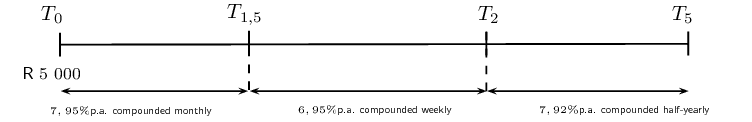Home Practice
For learners and parents For teachers and schools
Textbooks
Full catalogue
Pricing SupportLog in

We think you are located in United States. Is this correct?

# 9.3 Timelines

## 9.3 Timelines (EMBJK)

Interest can be compounded more than once a year. For example, an investment can be compounded monthly or quarterly. Below is a table of compounding terms and their corresponding numeric value ($$p$$). When amounts are compounded more than once per annum, we multiply the number of years by $$p$$ and we also divide the interest rate by $$p$$.

 Term $$p$$ yearly / annually $$\text{1}$$ half-yearly / bi-annually $$\text{2}$$ quarterly $$\text{4}$$ monthly $$\text{12}$$ weekly $$\text{52}$$ daily $$\text{365}$$
temp text

## Worked example 12: Timelines

$$\text{R}\,\text{5 500}$$ is invested for a period of $$\text{4}$$ years in a savings account. For the first year, the investment grows at a simple interest rate of $$\text{11}\%$$ p.a. and then at a rate of $$\text{12,5}\%$$ p.a. compounded quarterly for the rest of the period. Determine the value of the investment at the end of the $$\text{4}$$ years.

### Draw a timeline and write down known variables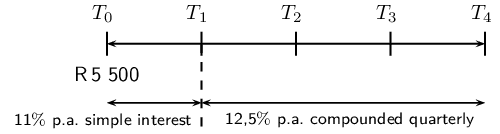In the timeline above, the intervals are given in years. For example, $$T_0$$ is the start of the investment, $$T_1$$ is the end of the first year and $$T_4$$ is the end of the fourth year.

### Use the simple interest formula to calculate $$A$$ at $$T_1$$

\begin{align*} A &= P(1 + in) \\ &= \text{5 500}(1 + \text{0,11}) \\ &= \text{R}\,\text{6 105} \end{align*}

### Use the compound interest formula to calculate $$A$$ at $$T_4$$

The investment is compounded quarterly, therefore:

\begin{align*} n &= 3 \times 4 \\ &= 12 \\ \text{and } i &= \frac{\text{0,125}}{4} \end{align*}

Also notice that the accumulated amount at the end of the first year becomes the principal amount at the beginning of the second year.

\begin{align*} A &= P(1 + i)^n \\ &= \text{6 105} \left( 1 + \frac{\text{0,125}}{4} \right)^{12} \\ &= \text{R}\,\text{8 831,88} \end{align*}

The value of the investment at the end of the $$\text{4}$$ years is $$\text{R}\,\text{8 831,88}$$.

temp text

## Worked example 13: Timelines

$$\text{R}\,\text{150 000}$$ is deposited in an investment account for a period of $$\text{6}$$ years at an interest rate of $$\text{12}\%$$ p.a. compounded half-yearly for the first $$\text{4}$$ years and then $$\text{8,5}\%$$ p.a. compounded yearly for the rest of the period. A deposit of $$\text{R}\,\text{8 000}$$ is made into the account after the first year and then another deposit of $$\text{R}\,\text{2 000}$$ is made $$\text{5}$$ years after the initial investment. Calculate the value of the investment at the end of the $$\text{6}$$ years.

### Draw a timeline and write down known variables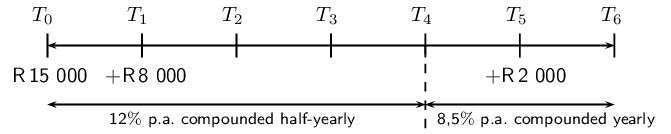Remember to show when the additional deposits of $$\text{R}\,\text{8 000}$$ and $$\text{R}\,\text{2 000}$$ where made into the account. It is very important to note that the interest rate changes at $$T_4$$.

We break this question down into parts and consider each amount separately.

### The initial deposit at $$T_0$$

Between $$T_0$$ and $$T_4$$:

We notice that interest for the first $$\text{4}$$ years is compounded half-yearly, therefore:

\begin{align*} n_1 &= 4 \times 2 \\ &= 8 \\ \text{and } i_1 &= \frac{\text{0,12}}{2} \end{align*}

Between $$T_4$$ and $$T_6$$:

\begin{align*} n_2 &= 2 \\ \text{and } i_2 &= \text{0,085} \end{align*}

Therefore the total growth of the initial deposit over the $$\text{6}$$ years is:

\begin{align*} A &= P(1 + i_1)^{n_1}(1 + i_2)^{n_2} \\ &= \text{150 000} \left( 1 + \frac{\text{0,12}}{2} \right)^8 \left(1 + \text{0,085}\right)^2 \end{align*}

### The deposit at $$T_1$$

Between $$T_1$$ and $$T_4$$:

Interest on this deposit is compounded half-yearly for $$\text{3}$$ years, therefore:

\begin{align*} n_3 &= 3 \times 2 \\ &= 6 \\ \text{and } i_3 &= \frac{\text{0,12}}{2} \end{align*}

Between $$T_4$$ and $$T_6$$:

\begin{align*} n_4 &= 2 \\ \text{and } i_4 &= \text{0,085} \end{align*}

Therefore the total growth of the deposit over the $$\text{5}$$ years is:

\begin{align*} A &= P(1 + i_3)^{n_3}(1 + i_4)^{n_4} \\ &= \text{8 000} \left(1 + \frac{\text{0,12}}{2} \right)^6 \left(1 + \text{0,085}\right)^2 \end{align*}

### The deposit at $$T_5$$

Accumulate interest for only $$\text{1}$$ year:

\begin{align*} A &= P(1 + i)^n \\ &= \text{2 000}(1 + \text{0,085})^1 \end{align*}

### Determine the total calculation

To get as accurate an answer as possible, we do the the calculation on the calculator in one step. Using the memory and answer recall function on the calculator, we avoid rounding off until we get the final answer.

\begin{align*} A &= \text{150 000} \left( 1 + \frac{\text{0,12}}{2} \right)^8 (1 + \text{0,085})^2 + \text{8 000} \left( 1 + \frac{\text{0,12}}{2} \right)^6 (1 + \text{0,085})^2 + \text{2 000}(1 + \text{0,085})^1 \\ &= \text{R}\,\text{296 977,00} \end{align*}

The value of the investment at the end of the $$\text{6}$$ years is $$\text{R}\,\text{296 977,00}$$.

temp text

## Worked example 14: Timelines

$$\text{R}\,\text{60 000}$$ is invested in an account which offers interest at $$\text{7}\%$$ p.a. compounded quarterly for the first $$\text{18}$$ months. Thereafter the interest rate changes to $$\text{5}\%$$ p.a. compounded monthly. Three years after the initial investment, $$\text{R}\,\text{5 000}$$ is withdrawn from the account. How much will be in the account at the end of $$\text{5}$$ years?

### Draw a timeline and write down known variables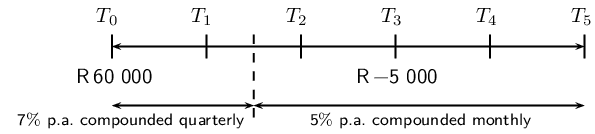Remember to show when the withdrawal of $$\text{R}\,\text{5 000}$$ was taken out of the account. It is also important to note that the interest rate changes after $$\text{18}$$ months ($$T_{1\frac{1}{2}}$$).

We break this question down into parts and consider each amount separately.

### The initial deposit at $$T_0$$

Interest for the first $$\text{1,5}$$ years is compounded quarterly, therefore:

\begin{align*} n_1 &= \text{1,5} \times 4 \\ &= 6 \\ \text{and } i_1 &= \frac{\text{0,07}}{4} \end{align*}

Interest for the remaining $$\text{3,5}$$ years is compounded monthly, therefore:

\begin{align*} n_2 &= \text{3,5} \times 12 \\ &= 42 \\ \text{and } i_2 &= \frac{\text{0,05}}{12} \end{align*}

Therefore the total growth of the initial deposit over the $$\text{5}$$ years is:

\begin{align*} A &= P(1 + i_1)^{n_1} (1 + i_2)^{n_2} \\ &= \text{60 000} \left( 1 + \frac{\text{0,07}}{4} \right)^6 \left(1 + \frac{\text{0,05}}{12} \right)^{42} \end{align*}

### The withdrawal at $$T_3$$

We calculate the interest that the $$\text{R}\,\text{5 000}$$ would have earned if it had remained in the account:

\begin{align*} n &= 2 \times 12 \\ &= 24 \\ \text{and } i &= \frac{\text{0,05}}{12} \end{align*}

Therefore we have that:

\begin{align*} A &= P(1 + i)^n \\ &= \text{5 000} \left(1 + \frac{\text{0,05}}{12} \right)^{24} \end{align*}

### Determine the total calculation

We subtract the withdrawal and the interest it would have earned from the accumulated amount at the end of the $$\text{5}$$ years:

\begin{align*} A &= \text{60 000} \left( 1 + \frac{\text{0,07}}{4} \right)^6 \left(1 + \frac{\text{0,05}}{12}\right)^{42} - \text{5 000} \left(1 + \frac{\text{0,05}}{12} \right)^{24} \\ &= \text{R}\,\text{73 762,19} \end{align*}

The value of the investment at the end of the $$\text{5}$$ years is $$\text{R}\,\text{73 762,19}$$.

## Timelines

Textbook Exercise 9.5

After a $$\text{20}$$-year period Josh's lump sum investment matures to an amount of $$\text{R}\,\text{313 550}$$. How much did he invest if his money earned interest at a rate of $$\text{13,65}\%$$ p.a. compounded half yearly for the first $$\text{10}$$ years, $$\text{8,4}\%$$ p.a. compounded quarterly for the next five years and $$\text{7,2}\%$$ p.a. compounded monthly for the remaining period?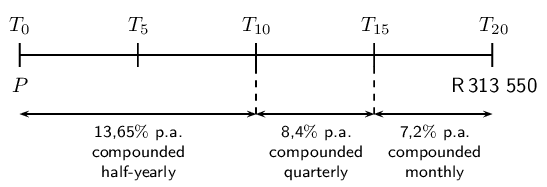Sindisiwe wants to buy a motorcycle. The cost of the motorcycle is $$\text{R}\,\text{55 000}$$. In $$\text{1 998}$$ Sindisiwe opened an account at Sutherland Bank with $$\text{R}\,\text{16 000}$$. Then in $$\text{2 003}$$ she added $$\text{R}\,\text{2 000}$$ more into the account. In $$\text{2 007}$$ Sindisiwe made another change: she took $$\text{R}\,\text{3 500}$$ from the account. If the account pays $$\text{6}\%$$ p.a. compounded half-yearly, will Sindisiwe have enough money in the account at the end of $$\text{2 012}$$ to buy the motorcycle?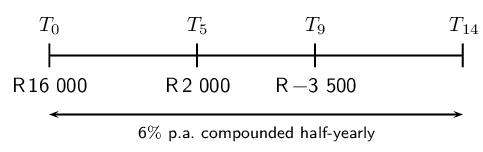A loan has to be returned in two equal semi-annual instalments. If the rate of interest is $$\text{16}\%$$ per annum, compounded semi-annually and each instalment is $$\text{R}\,\text{1 458}$$, find the sum borrowed.

\begin{align*} A &= P(1 + i)^n \\ &= P_1(1 + \frac{\text{0,16}}{2})^1 \\ &= P_1(\text{1,08}) \\ \text{Therefore } P_1(\text{1,08}) &= \text{1 458} + P_2 \\ \text{and } P_2(\text{1,08})&= \text{1 458} \\ \therefore P_2 &= \frac{\text{1 458}}{\text{1,08}} \\ \therefore P_1(\text{1,08}) &= \text{1 458} + P_2 \\ \therefore P_1 &= \frac{\text{1 458}}{\text{1,08}}+ \frac{\text{1 458}}{(\text{1,08})^2} \\ &=\text{R}\,\text{2 600} \end{align*}

A man named Phillip invests $$\text{R}\,\text{10 000}$$ into an account at North Bank at an interest rate of $$\text{7,5}\%$$ p.a. compounded monthly. After $$\text{5}$$ years the bank changes the interest rate to $$\text{8}\%$$ p.a. compounded quarterly. How much money will Phillip have in his account $$\text{9}$$ years after the original deposit?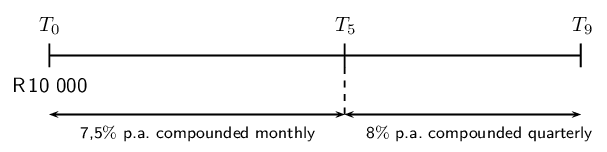$$\text{R}\,\text{75 000}$$ is invested in an account which offers interest at $$\text{11}\%$$ p.a. compounded monthly for the first $$\text{24}$$ months. Then the interest rate changes to $$\text{7,7}\%$$ p.a. compounded half-yearly. If $$\text{R}\,\text{9 000}$$ is withdrawn from the account after one year and then a deposit of $$\text{R}\,\text{3 000}$$ is made three years after the initial investment, how much will be in the account at the end of $$\text{6}$$ years?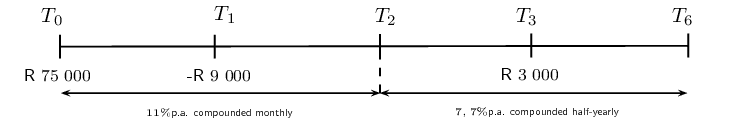Christopher wants to buy a computer, but right now he doesn't have enough money. A friend told Christopher that in $$\text{5}$$ years the computer will cost $$\text{R}\,\text{9 150}$$. He decides to start saving money today at Durban United Bank. Christopher deposits $$\text{R}\,\text{5 000}$$ into a savings account with an interest rate of $$\text{7,95}\%$$ p.a. compounded monthly. Then after $$\text{18}$$ months the bank changes the interest rate to $$\text{6,95}\%$$ p.a. compounded weekly. After another $$\text{6}$$ months, the interest rate changes again to $$\text{7,92}\%$$ p.a. compounded two times per year. How much money will Christopher have in the account after $$\text{5}$$ years, and will he then have enough money to buy the computer?